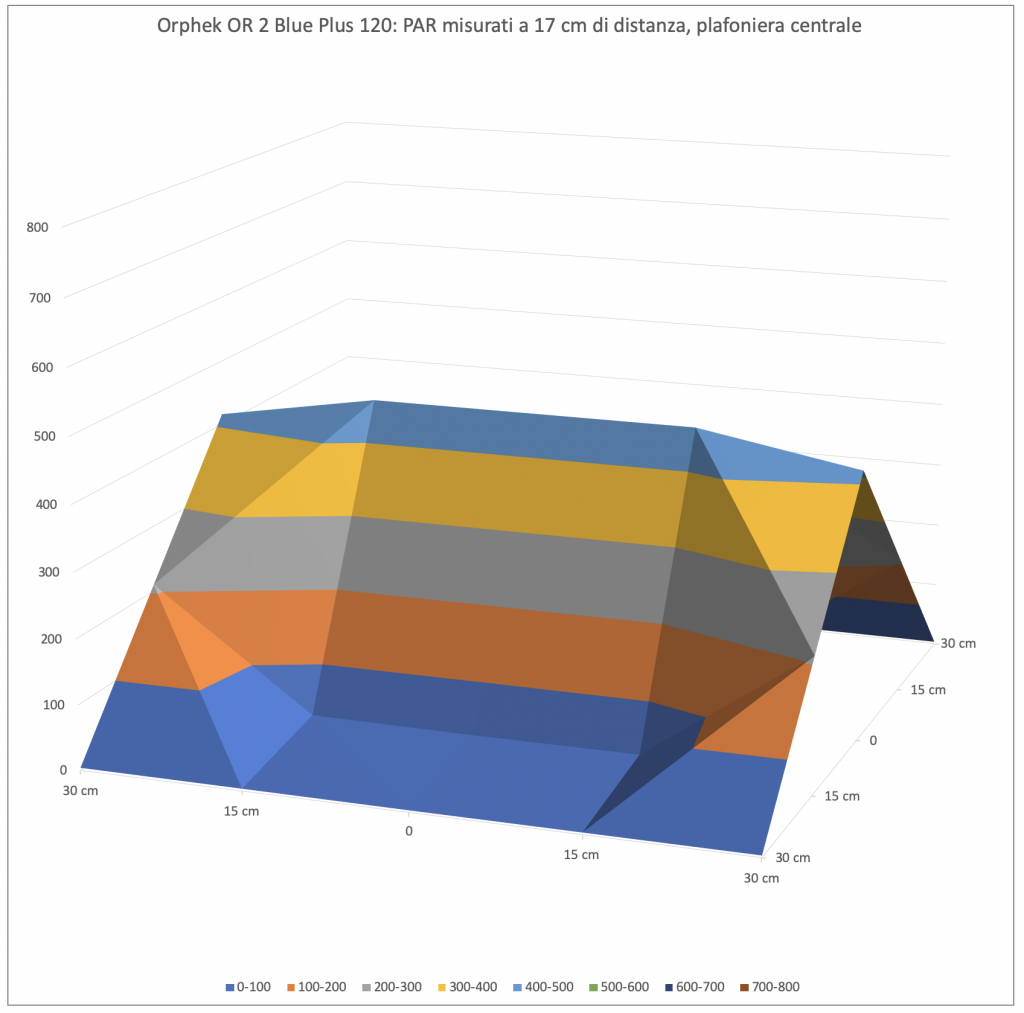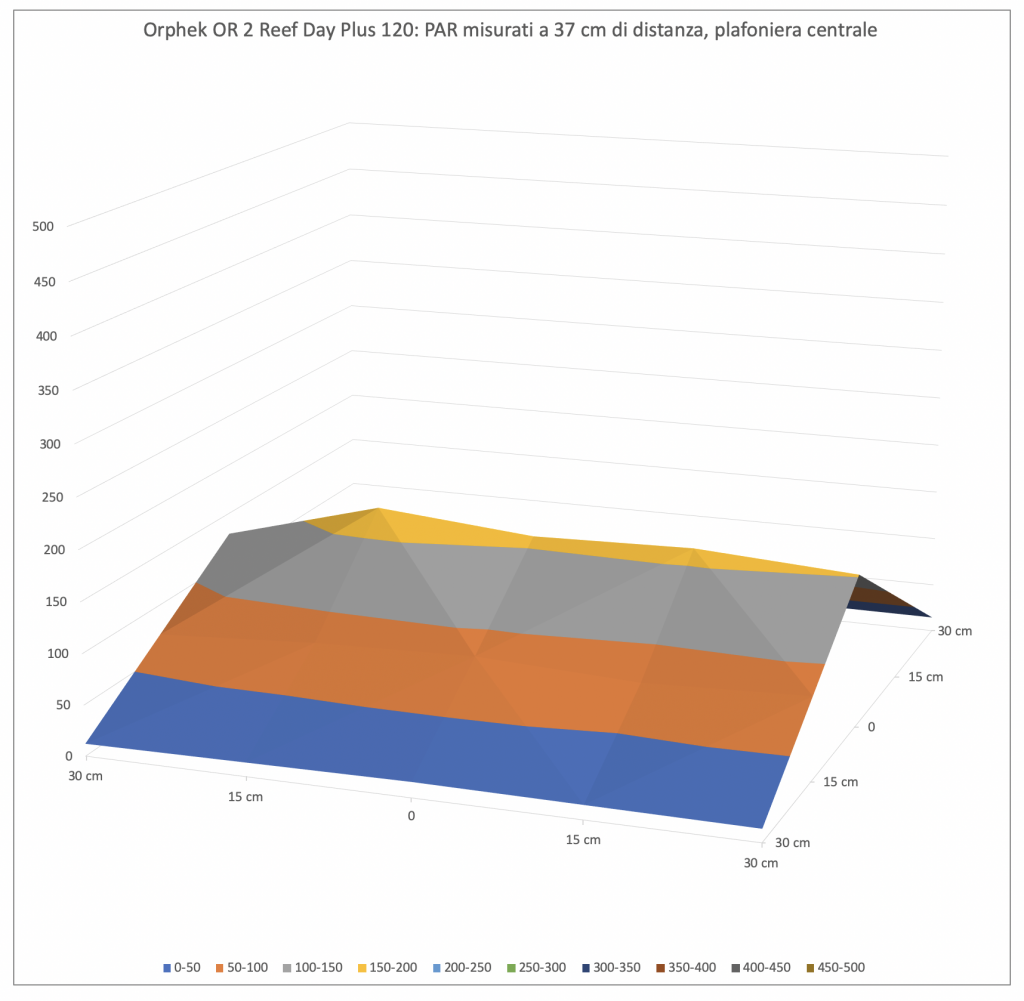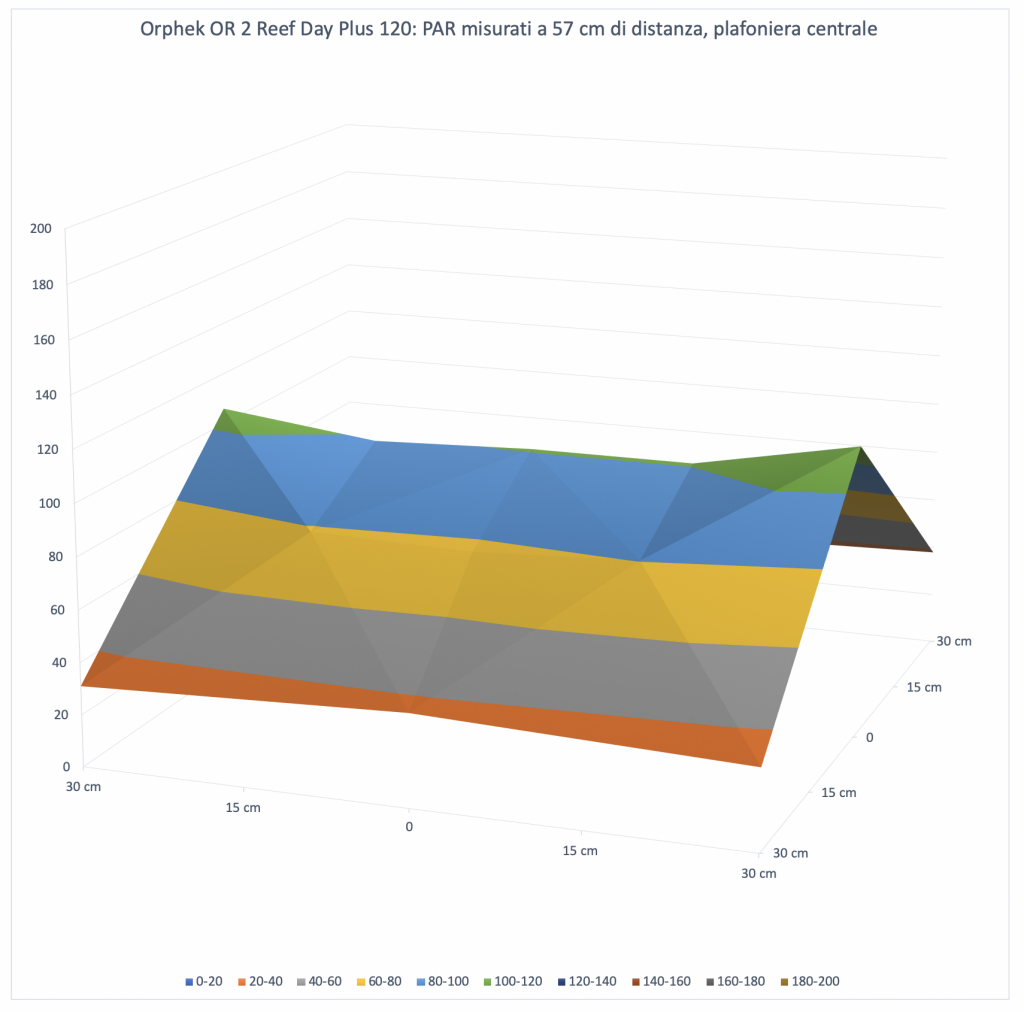# LED bars Orphek OR2 Blue Plus tested in our DaniReef LAB

## Our new method DaniReef LAB for PAR measurement

During the long nights spent on our forum we always wondered how could we compare PAR from different ceiling lights. Even though we had the perfect device, the Quantum Meter MQ-510 di Apogee, we always referred only to the value measured at the centre at 20 cm of distance, more or less.

The Quantum Meter MQ-510 measures the PAR, expressed in PFFD that is photosynthetic photon flux density in μmol m-2 s-1. This device is calibrated to work underwater, so if it’s in air the measured value has to be divided by 1,32 that’s the dive factor. We have to do the conversion. The values you’re going to see are correct.

We decided to make a square base of 70×70 cm, we set 17 fiducial points where we placed the sensor Quantum Meter MQ-510 and we also made 3 lifts of 20, 40 and 60 cm for the ceiling light, in order to have the same distance from the sensor. This will allow us to create the curves which can be compared to other ceiling lights’s, all tested at the same distances. Notice that this distance is measured between the base of the sensor and the ceiling light. In reality it should be decreased of 3,5 cm that is the height of the measurement cylinder and increased by 0,5 cm that’s the dimension of the spacers that lift the ceiling light.

In total the three measurements are made from 17, 37 and 57 cm of distance. Because they’ve been done in air they will be corrected.

In truth, the bars gave us other problems, because we had to distance the spacers in order to consider the light coming from the external LED.

## PAR measurement from 17 cm of distance

This is the condition of the test. Below there are the chart and the device, above you can glimpse the bar placed on the spacers.

These are the values:

And that’s the corresponding chart, we came back to our classical scale in order to compare similar ceiling lights, but being this LED bars we lowered the maximum scale. For the future bars you will find the same evaluation scale.

As you can see the bar created a curve that looks like a tent, with the spacial differencies due to the kind of LED. Unlike the classic ceiling lights the decay is very linear, at 30 cm from the ligh source.

Here are the two charts of the two LED bars.Orphek OR2 Blue Plus

Surprisingly, the PAR are higher in the Blue Plus version. The scale, obviously, is the same.

## PAR measurement from 37 cm of distance

This is the condition of the test. Below there are the chart and the device, above the bar placed on the spacers.

Here the collected values:

And that’s the corresponding chart.

With a bigger space between ceiling lights and sensor, it decreases the difference between PAR in the middle and on the sides. The light spreads, but the central value decreases from 459 to 207 μmol m-2 s-1, measured in the brighter spots.

It stands out that the covering is wider.Orphek OR2 Reef Day Plus

Even in this case, as we expected, the Blue Plus bar shows a higher number of PAR. Moreover, using LED with comparable wavelength the charts are way more linear.

## PAR measurement from 57 cm of distance

This is the condition of the test. Below there’s the chart, from this distance the device is very visible, and the bar placed on the spacers. Even visually the illuminated area is wider, so the specific power per cm decreases.

Here are the collected values:

And that’s the corresponding chart.

Increasing even more the space between ceiling light and sensor, the difference between the PAR in the middle and on the sides doesn’t decrease as we did expected but it increases instead. The value in the middle decreases again from 459 to 207 to 130 μmol m-2 s-1.Orphek OR2 Reef Day Plus

The difference between the covering of the two bars increases even more, especially in the middle, while on the sides it’s pretty much the same.

## The PAR in the middle in the different configurations

Let’s continue with our technical measurements. We can see how, in a chart, the PAR collected in the middle decrease in the three different distances of the tests.

## Energy variation of the LED bars Orphek OR2 Blue Plus depending on the distances

This is the most significant and most comparable data. The energy variation. We calculated the volumes of the three surfaces previously seen. It’s obvious that moving away from the ceiling light the PAR decrease, also because the light illuminates a wider space. We try to consider all the light energy in this square of 60 cm under the bar. And it’s visible that the three values of 323.000, 291.000 and 259.000 assume a different connotation compareed to the values in the middle. When the first ones decrease because the distances increase, the subtended area, that is the energy, decreases very slowly. The three areas are enough similar. Little energy is lost over the area.

Considering the values of the three curves you can see that on the sides there’s more light at 57 cm rather than 37.

Let’s continue on page three with the the costs, the comparison with other ceiling lights and our final opinion.